# Newton’s third law of motionPage 5

#### WATCH ALL SLIDES

Whenever one object exerts a force on a second object, the second object exerts an equal and opposite force on the first.

Slide 32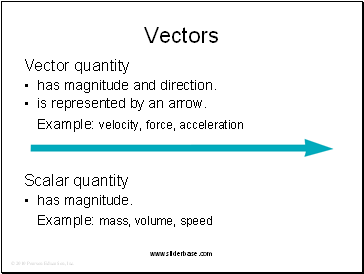## Vectors

Vector quantity

has magnitude and direction.

is represented by an arrow.

Example: velocity, force, acceleration

Scalar quantity

has magnitude.

Example: mass, volume, speed

Slide 33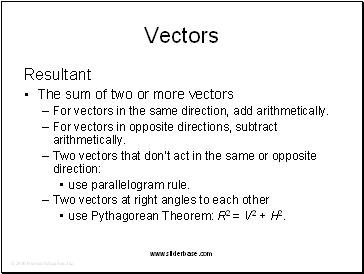Vectors

Resultant

The sum of two or more vectors

For vectors in the same direction, add arithetically.

For vectors in opposite directions, subtract arithetically.

Two vectors that don’t act in the same or opposite direction:

use parallelogram rule.

Two vectors at right angles to each other

use Pythagorean Theorem: R2 = V2 + H2.

Slide 34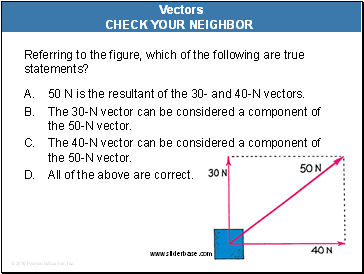Referring to the figure, which of the following are true statements?

A. 50 N is the resultant of the 30- and 40-N vectors.

The 30-N vector can be considered a component of the 50-N vector.

The 40-N vector can be considered a component of the 50-N vector.

All of the above are correct.

Vectors

CHECK YOUR NEIGHBOR

Slide 35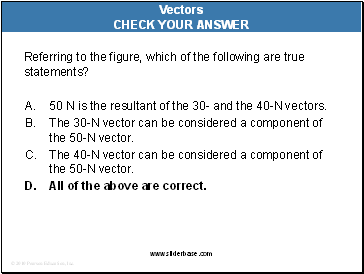Referring to the figure, which of the following are true statements?

A. 50 N is the resultant of the 30- and the 40-N vectors.

The 30-N vector can be considered a component of the 50-N vector.

The 40-N vector can be considered a component of the 50-N vector.

All of the above are correct.

Vectors

CHECK YOUR ANSWER

Slide 36Referring to the figure, which of the following are true statements?

A. 100 km/h is the resultant of the 80- and 60-km/h vectors.

The 80-km/h vector can be considered a component of the 100-km/h vector.

C. The 60-km/h vector can be considered a component of the 100-km/h vector.

All of the above are correct.

Vectors

CHECK YOUR NEIGHBOR

Slide 37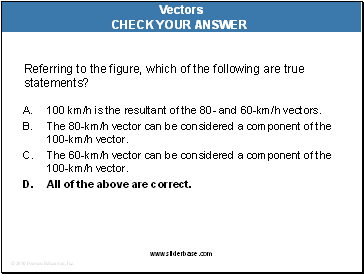Referring to the figure, which of the following are true statements?

A. 100 km/h is the resultant of the 80- and 60-km/h vectors.

The 80-km/h vector can be considered a component of the 100-km/h vector.

C. The 60-km/h vector can be considered a component of the 100-km/h vector.

All of the above are correct.

Vectors

CHECK YOUR ANSWER

Slide 38Vectors

Vector components

Vertical and horizontal components of a vector are perpendicular to each othe.r

Determined by resolution.

Slide 39

Go to page:
1  2  3  4  5  6

## Last added presentations

© 2010-2019 powerpoint presentations